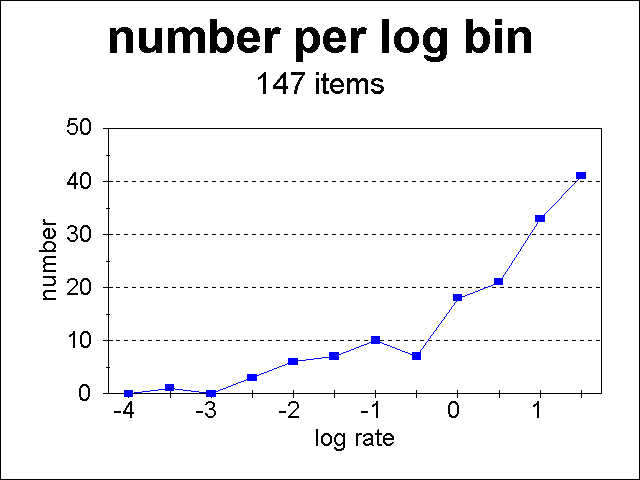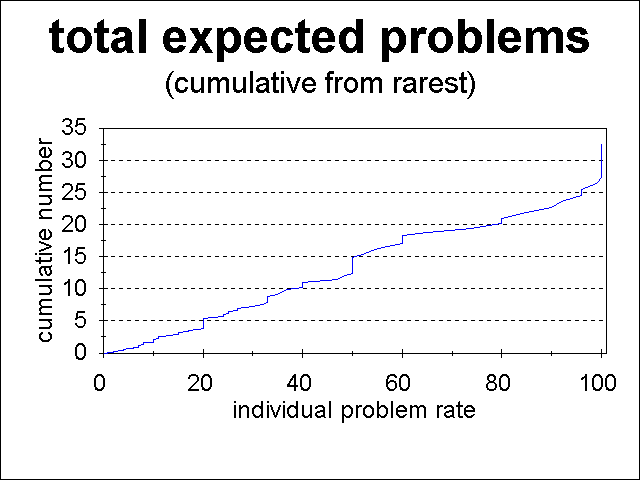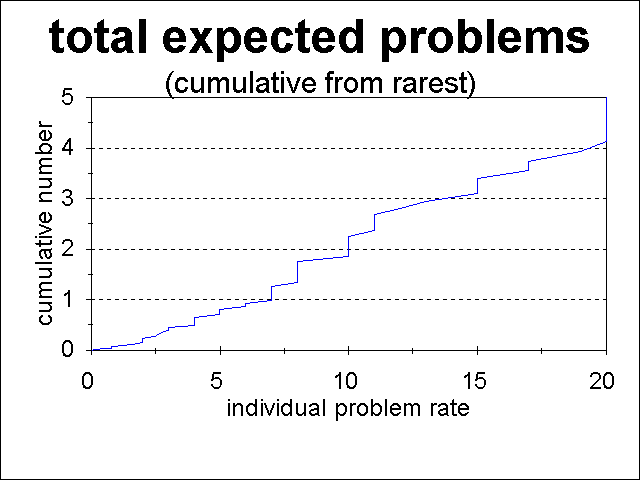# Analysis of Rate of Human Problems

Humans are afflicted with a large number of diseases and other problems. There are several interesting questions related to the occurrence rate of these problems:

For some years now, I have been collecting estimates of the Rate of Human Diseases, Problems and Conditions. I define the "occurrence rate" for a given problem as the percent of people who are affected at least once in their lifetime by that problem. Now that I've put 147 problems into a spreadsheet, I can do some preliminary analysis to begin to answer these questions.

Several caveats should be kept in mind:

• The dataset I am analyzing is undoubtedly seriously incomplete, since I myself have a backlog of probably 100 other problems to add to my database, and I probably don't have a complete list yet of problems to add.
• There are undoubtedly biases in the reporting rates for different disease. For example, newspapers may be biased to report the "rare" and therefore more interesting problems at a higher rate than "common" problems. On the other hand, people may find it of more interest to read about "their" problems, and thus there may be a bias toward reporting common problems more frequently.
For a list of problems that can affect individual rate estimates, see the Rate of Human Diseases, Problems and Conditions. Since the analysis here is statistical, the uncertainties of individual rates are much less important.

I will continue to add problems, and when I accumulate a significantly expanded set, will revisit this analysis.

## Total Number of Rare Problems Compared To the Number of Common Problems

With those caveats in mind, the following plot shows the number of human problems plotted versus logarithmic rate bins. Each bin is 0.5 wide in log10 {rate in %}, and hence contains problems that have the same rate within a factor of 3.16. The first bin on the right, with the largest number of problems, contains all problems with rates of 32% to 100% (log rates of 1.5 to 2.0).There are significantly more problems that occur at high rates than ones that occur at low rates. There are several probable reasons for this:

• It is probably difficult for infectious diseases to be rare. If they are so weak that they can only infect a small percentage of humans, they are likely to die out in a relatively short time. Thus most infectious diseases in my list, such as colds, flu, sinus infections, salmonella, etc., have rates much closer to 100% than to 1%.

• Many human problems caused by aging and lifestyles must also be common since the basic design of humans is pretty much the same and our lifestyles are pretty similar. Thus heart attacks, cancer, presbyopia, arthritis, etc. are quite common because that's what our bodies do as they get older. Dog bites, zoonotic diseases, etc. are quite common because humans have chosen to have a large number of pets and other lifestyles that create such problems.

• Rare problems, on the other hand, tend to be genetic problems such as PKU, birth defects, long QT syndrome, Huntington's disease, fragile X syndrome, etc., although there are a few rare infectious diseases such as the notorious flesh-eating bacteria. Thus most of the rare problems arise from the mathematics and population dynamics of the equilibrium level of such genetic problems. I am no expert, but I believe that it is the case that such genetic problems can never be very common in an equilibrium level. (I'd love to be enlightened from any expert reading this!)

## What Is the Rarest Disease You Should Expect to Have?

If there are 100 problems that occur at the rate of 1%, then you should expect to suffer one of those problems on average. If I add up the rates of problems, starting from the rarest problem, the point at which the rate gets to 100% tells me that I should expect to suffer one problem whose rate is below that point.

This sounds like a mouthful, but it is a simple concept. Suppose that this is the entire universe of problems and their rates:

• There are only 10 problems that each occur at a rate of 1%;
• There are 15 problems that each occur at a rate of 2%; and
• There are 20 problems that each occur at a rate of 3%.
• Any distribution of problems that each occur with higher rates.
Then:
• 10% of people will each have a problem that occurs in only 1% of people (since there are 10 different problems each at a rate of 1%);
• 40% of people will have a problem that occurs in 2% of people or less (since 10% have "1% problems" and 30% have "2% problems"); and
• 100% of people will have a problem that occurs in only 3% of people or less;
Thus on average everyone will have one of these 45 problems, even though each problem occurs in no more than 3% of the entire population. (See Technical Footnote if you are a probability expert.)

Hence the answer in this case is that on average every person in that hypothetical universe would expect to suffer from at least one problem that occurs in no more than 3% of the population. This is true no matter how many problems there are that are more common, since one simply adds up the total population rate starting from the least common diseases, to answer my question.

The following two plots give the cumulative rate of human problems plotted versus the rate of the last problem added to the cumulative total, with the second plot simply having an expanded scale to see where the cumulative rate equals 1:The rate at which the cumulative rate is 1.00 gives the answer to the question. In this case, the cumulative rate reaches 1.00 at a rate of 7%. Thus every human should expect to have at least one disease which occurs in 7% or less of the general population.

Because my list of problems is incomplete, the answer of "a rate of 7%" is an upper limit, and it is likely that the true answer is a rate well under 7%. As I add more problems to my list, I'll explore how that rate decreases.

Note that on average a person should suffer from 33 of the problems in my list! Sigh......

## Technical Footnote re Probability

My calculation actually gives the expected rate of problems in a population, and not the probability that the entire population suffers at least one problem. Technically, 36% of that population will not suffer at least one of these problems since the correct way to calculate the rate of the population with those problem rates is to calculate the probability that a given person will have none of those problems. That probability is:

(1-.01)10 * (1-.02)15 * (1-.03)20 = 0.36.

In the interests of simplicity, I have neglected the difference between 0.64 and 1.00! This neglect simply reinforces the fact that my calculation gives an upper limit to the answer.

Go To: